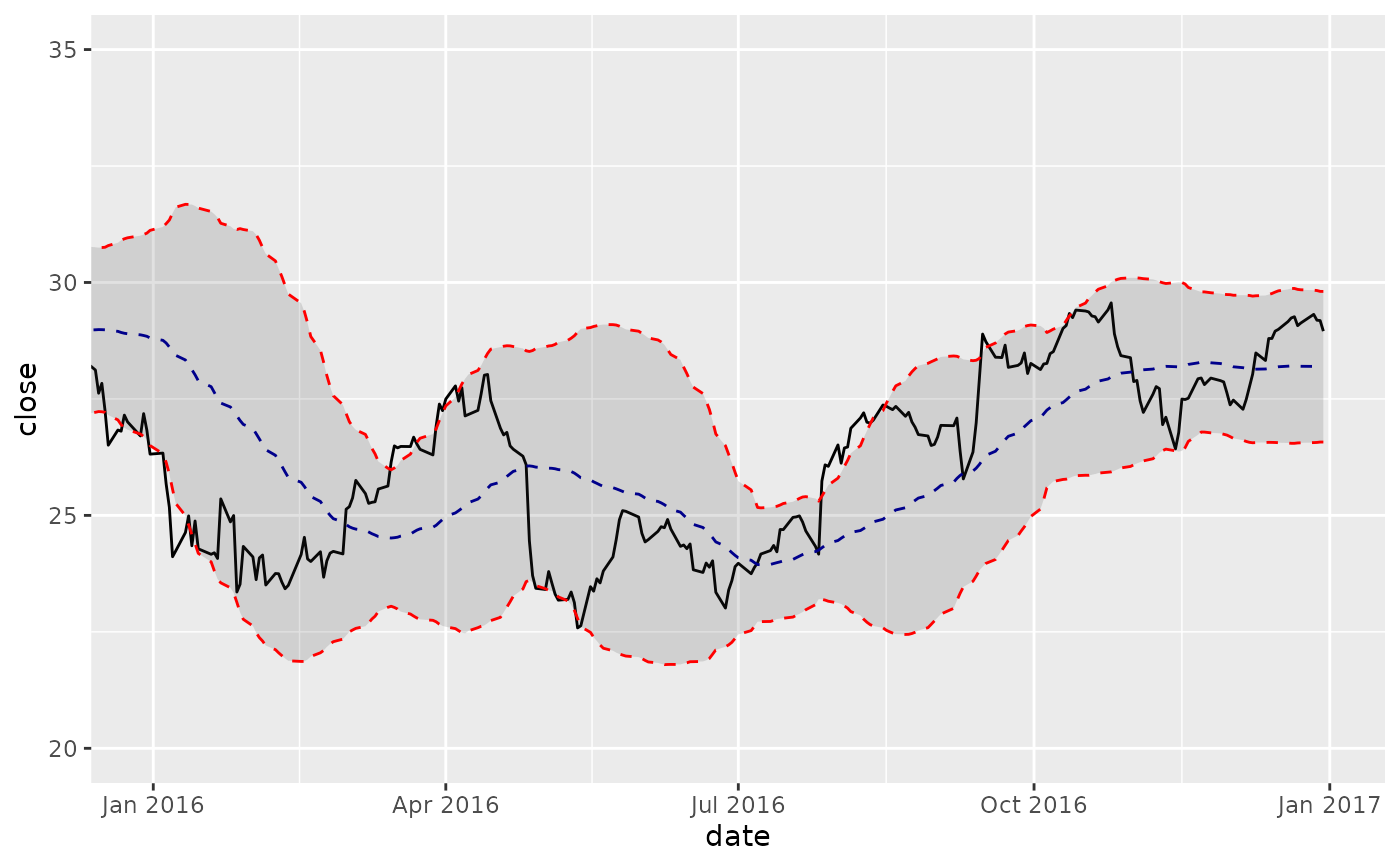Bollinger Bands plot a range around a moving average typically two standard deviations up and down. The geom_bbands() function enables plotting Bollinger Bands quickly using various moving average functions. The moving average functions used are specified in TTR::SMA() from the TTR package. Use coord_x_date() to zoom into specific plot regions. The following moving averages are available:

geom_bbands(
mapping = NULL,
data = NULL,
position = "identity",
na.rm = TRUE,
show.legend = NA,
inherit.aes = TRUE,
ma_fun = SMA,
n = 20,
sd = 2,
wilder = FALSE,
ratio = NULL,
v = 1,
wts = 1:n,
color_ma = "darkblue",
color_bands = "red",
alpha = 0.15,
fill = "grey20",
...
)

geom_bbands_(
mapping = NULL,
data = NULL,
position = "identity",
na.rm = TRUE,
show.legend = NA,
inherit.aes = TRUE,
ma_fun = "SMA",
n = 10,
sd = 2,
wilder = FALSE,
ratio = NULL,
v = 1,
wts = 1:n,
color_ma = "darkblue",
color_bands = "red",
alpha = 0.15,
fill = "grey20",
...
)

## Arguments

mapping Set of aesthetic mappings created by ggplot2::aes() or ggplot2::aes_(). If specified and inherit.aes = TRUE (the default), it is combined with the default mapping at the top level of the plot. You must supply mapping if there is no plot mapping. The data to be displayed in this layer. There are three options: If NULL, the default, the data is inherited from the plot data as specified in the call to ggplot2::ggplot(). A data.frame, or other object, will override the plot data. All objects will be fortified to produce a data frame. See ggplot2::fortify() for which variables will be created. A function will be called with a single argument, the plot data. The return value must be a data.frame., and will be used as the layer data. Position adjustment, either as a string, or the result of a call to a position adjustment function. If TRUE, silently removes NA values, which typically desired for moving averages. logical. Should this layer be included in the legends? NA, the default, includes if any aesthetics are mapped. FALSE never includes, and TRUE always includes. It can also be a named logical vector to finely select the aesthetics to display. If FALSE, overrides the default aesthetics, rather than combining with them. This is most useful for helper functions that define both data and aesthetics and shouldn't inherit behaviour from the default plot specification, e.g. ggplot2::borders(). The function used to calculate the moving average. Seven options are available including: SMA, EMA, WMA, DEMA, ZLEMA, VWMA, and EVWMA. The default is SMA. See TTR::SMA() for underlying functions. Number of periods to average over. Must be between 1 and nrow(x), inclusive. The number of standard deviations to use. logical; if TRUE, a Welles Wilder type EMA will be calculated; see notes. A smoothing/decay ratio. ratio overrides wilder in EMA, and provides additional smoothing in VMA. The 'volume factor' (a number in [0,1]). See Notes. Vector of weights. Length of wts vector must equal the length of x, or n (the default). Select the line color to be applied for the moving average line and the Bollinger band line. Used to adjust the alpha transparency for the BBand ribbon. Used to adjust the fill color for the BBand ribbon. Other arguments passed on to ggplot2::layer(). These are often aesthetics, used to set an aesthetic to a fixed value, like color = "red" or size = 3. They may also be parameters to the paired geom/stat.

## Aesthetics

The following aesthetics are understood (required are in bold):

• x, Typically a date

• high, Required to be the high price

• low, Required to be the low price

• close, Required to be the close price

• volume, Required for VWMA and EVWMA

• colour, Affects line colors

• fill, Affects ribbon fill color

• alpha, Affects ribbon alpha value

• group

• linetype

• size

See individual modeling functions for underlying parameters:

• TTR::SMA() for simple moving averages

• TTR::EMA() for exponential moving averages

• TTR::WMA() for weighted moving averages

• TTR::DEMA() for double exponential moving averages

• TTR::ZLEMA() for zero-lag exponential moving averages

• TTR::VWMA() for volume-weighted moving averages

• TTR::EVWMA() for elastic, volume-weighted moving averages

• coord_x_date() for zooming into specific regions of a plot

## Examples

# Load libraries
library(tidyquant)
library(dplyr)
library(ggplot2)

AAPL <- tq_get("AAPL", from = "2013-01-01", to = "2016-12-31")

# SMA
AAPL %>%
ggplot(aes(x = date, y = close)) +
geom_line() +           # Plot stock price
geom_bbands(aes(high = high, low = low, close = close), ma_fun = SMA, n = 50) +
coord_x_date(xlim = c(as_date("2016-12-31") - dyears(1), as_date("2016-12-31")),
ylim = c(75, 125))# EMA
AAPL %>%
ggplot(aes(x = date, y = close)) +
geom_line() +           # Plot stock price
geom_bbands(aes(high = high, low = low, close = close),
ma_fun = EMA, wilder = TRUE, ratio = NULL, n = 50) +
coord_x_date(xlim = c(as_date("2016-12-31") - dyears(1), as_date("2016-12-31")),
ylim = c(75, 125))# VWMA
AAPL %>%
ggplot(aes(x = date, y = close)) +
geom_line() +           # Plot stock price
geom_bbands(aes(high = high, low = low, close = close, volume = volume),
ma_fun = VWMA, n = 50) +
coord_x_date(xlim = c(as_date("2016-12-31") - dyears(1), as_date("2016-12-31")),
ylim = c(75, 125))